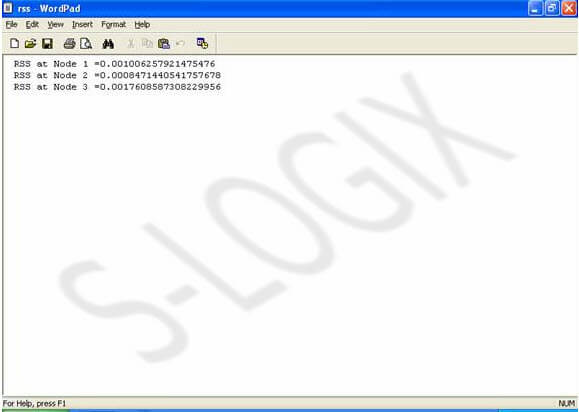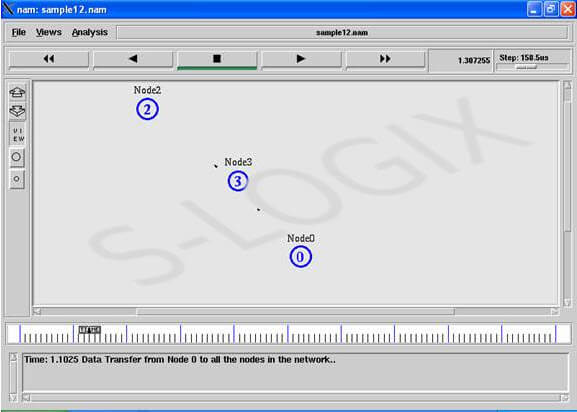• #5, First Floor, 4th Street Dr. Subbarayan Nagar Kodambakkam, Chennai-600 024 Landmark : Samiyar Madam
• pro@slogix.in
• +91- 81240 01111

How to calculate the Received Signal Strength (RSS) value of the node in ns2?

Description

Received Signal strength at the receiver node is calculated based on the distance of the receiver from the sender and transmission power of the signal at the sender node. Distance between nodes in wireless network is calculated. Distance output is printed in distance.tr file. Received Signal Strength is calculated and the result is printed in rss.tr file.

Sample Code

#Filename: sample12.tcl

set r [open distance.tr w]
#*****************************DISTANCE CALCULATION******************************************#
for {set i 0} {\$i < \$val(nn) } { incr i } {
puts "\n"
puts \$r "\n"

for {set j 0} {\$j < \$val(nn) } { incr j } {

set dx [expr \$xx(\$i) – \$xx(\$j)]
set dy [expr \$yy(\$i) – \$yy(\$j)]

set dx2 [expr \$dx * \$dx]
set dy2 [expr \$dy * \$dy]

set h2 [expr \$dx2 + \$dy2]

set h(\$i-\$j) [expr pow(\$h2, 0.5)]
puts "distance of node(\$i) from node(\$j) = \$h(\$i-\$j)"
puts \$r "distance of node(\$i) from node(\$j) h(\$i-\$j) = \$h(\$i-\$j)"

}
}

#***************************** RSS CALCULATION FROM Node 0 ******************************************#

set TransmitPower 0.281838

for {set i 1} {\$i < 10} {incr i} {